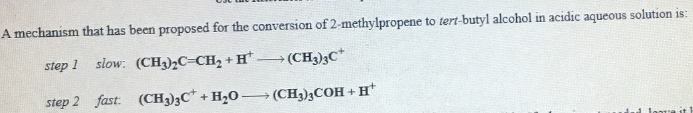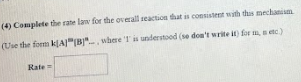# A mechanism that has been proposed for the conversion of 2-methylpropene to tert-butyl alcohol in acidic aqueous solution is: step 1 slow: (CH3)2C=CH2 + H^+ →(CH3)3C^+ step 2 fast: (CH303C^+ + H2O → (CH3)3COH + H^+ (4) Complete the rate law for the overall reaction that is consistent with this mechanism. (use the form k[A]^m[B]^n... , where '1' is understood (so don't write it) for m, n etc.)